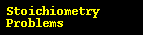# Molecular Formula 2

## Strategy:

1. Find the empirical formula.
• Get the mass of each element by assuming a certain overall mass for the sample (100 g is a good mass to assume when working with percentages).
• Convert the mass of each element to moles.
• Find the ratio of the moles of each element.
• Use the mole ratio to write the empirical fomula.

2. Find the mass of the empirical unit.

3. Figure out how many empirical units are in a molecular unit.

4. Write the molecular formula.

#### Show completed problemGen Chem Topic ReviewGeneral Chemistry Help Homepage